Next: Terzaghi's model Up: Alternative rock velocity models Previous: Alternative rock velocity models

## Biot's model

Biot's theory Bourbie et al. (1987) is based on the fundamental assumption of continuum mechanics that the wavelength is large in comparison with the size of the macroscopic elementary volume of the material and that the displacement is small so that the strain tensor is linear. These continuum conditions are readily satisfied by seismic studies. Furthermore, Biot assumes that the rock matrix is elastic and isotropic, that the liquid phase is continuous, that the rock matrix is solid, that the pores are disconnected, and that the porosity is isotropic and uniform. These assumptions are approximately true for many subsurface rocks.

The equation of continuity for a porous rock is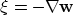whereis the local fluid increase due to the filtration of fluid. Ifis the displacement of the liquid phase andis the displacement of the solid phase, then the filtration velocity is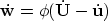where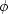is the rock porosity.

The constitutive equation is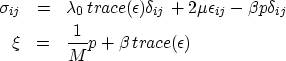(25) (26)
where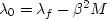.The coefficientis the standard shear modulus, which is independent of the fluid content. The coefficientis the second Lame coefficient for a closed rock sample - a rock sample filled with water and without any change in fluid contents (). Consequently,is linked to the elastic constants of the rock matrix and the fluid.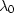is the analogue coefficient for an open system - a system with no external pressure (p = 0). Coefficient M is the pressure required to increase the fluid content of a unit volume at isovolumetric strain -.The coefficient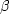quantifies the proportion of pressure due to the variation in fluid content for an open system. It is linked to the porosity and the geometry of the flow channels. All these coefficients can be measured in the laboratory on rock samples. In the limiting case that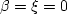and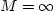we yield the constitutive relationship for a single solid phase.

The constitutive equation is derived from changes in the volumetric energy potential V due to infinitesimal increments in strain and fluid contents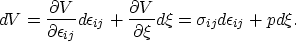where p is a pressure average in the fluid, sigma is the macroscopic stress tensor. The isotropy of the rock permits an approximation of the general tensor relation by the tensors first two invariants (the tensor's trace is the first invariant). Introduction of the standard Lame coefficients yields the constitutive relation above.

The constitutive equation at zero fluid pressure p = 0 and at hydrostatic fluid pressure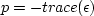yields relationships that when expressed in terms of the saturated bulk modulo Ks, the open or dry bulk modulo Kd, the bulk modulo of the fluid Kf, and the bulk modulo of the solid skeleton Km can be combined to the Gassmann equation 15. The equations assume quasi-static behaviour and are only true for low-frequency waves.

The equations of motion for porous rocks are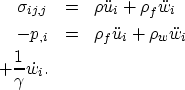(27) (28)
whereis the stress, u is the displacement of the matrix, w is the displacement of the fluid,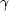is the effective transmissibility (or hydraulic permeability or fluid mobility). The transmissibility is the ratio of the absolute rock permeability k and the fluid viscosity: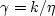.The average densityis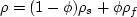. The effective fluid density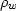takes the porosity and the shape of the pores into account. The coupling effect is usually described by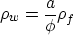where the tortuosity parameteris the factor incorporating the pore shape. Tortuosity tends to 1 as the porosity tends to 1. Berryman 1980 suggested to apply the tortuosity expression for solid spherical particles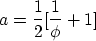to porous rock.

If there is no average relative fluid movement with respect to the overall macroscopic movement of the rock, i.e.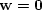, then the first equation of 28 is reduced to the standard single phase case. If the acceleration of the fluid and the rock are zero -- the motion is a steady state flow and the second equation of 28 reduces to Darcy's classic law of fluid flow in permeable material.

Bourlie 1987 derives The equations of motion from a strain potential expression that yields the constitutive equation, a potential expression for dissipation, and a potential expression for kinetic energy.

Biot's wave equation is, as usual, derived by substitution from the equation of motion 28 and the constitutive equation 26:(29)
where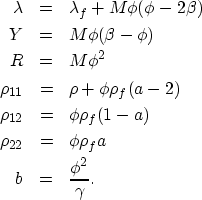(30)
b is the coefficient governing the wave's energy dissipation which in Biot's system is entirely due to relative motion of the fluid and matrix components.

The equations reduce to the limiting case of a perfect fluid when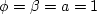,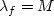, and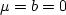(sincetends to infinity and the viscosity tends to ). Consequently, the first equation disappears of 30 and the second reduces to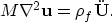If the medium is solid, we haveand a tends to infinity. Consequently, the second equation of 30 yields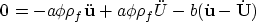while the first equation reduces toThe right-hand-side term of the two equations are equal. Consequently, the left hand expression of the second equation is zero, which is the standard wave equation for single phase solids.

Without Dissipation - b=0 which implies transmissibility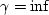or porosity- Biot's wave equation for porous rocks predicts two P-waves. For P-waves we can express the displacementsandas gradients of a potential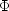:(31)
Consequently,and the wave equation expressions 30 reduce to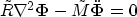where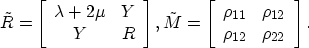The matrixis positive definite and consequently has two real eigenvalues vp1 and vp2. In the matrix's eigenvector reference system, the wave equation reduces to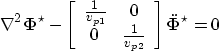where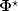is the potential function in the eigenvector reference system. The equation implies two decoupled P-waves. The second P-wave velocity vp2 is usually significantly smaller than the first. The faster vp1 merges with the classic P-wave in the absence of pore fluids.

Analogues introducing shear waves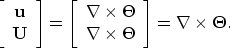(32)
we find a single shear wave with velocityThe fluid influences the shear velocity indirectly due to its effect on the overall inertia of the porous rock.

With dissipation the P-Wave ansatz 31 reduces the general wave equation 30 to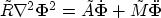(33)
whereis the damping matrix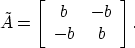The ansatz for harmonic P-waves is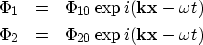(34) (35)
where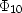and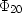are constant andand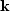are the angular frequency and the wave number. The introduction of this ansatz into the wave equationyields a system of two equation. To ensure that a solution exists the determinant of the homogeneous system has to be zero. This condition leads to dispersion relationships with two solutions: kP1 and kP2 which relate to the two types of P-waves found in the case without dissipation. Both wave numbers are complex. The real part describes the harmonic propagation: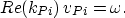where vPi relates to the velocities found in the non-dissipating discussion. The complex part governs the amplitude dissipation of the wave. The propagation velocity is dispersive since frequency dependent. As the frequency approaches zero, the source of dissipation - the relative motion between the fluid and the matrix - vanishes. At frequencies where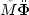can be ignored, the equation, once more, reduces to Darcy's law of steady flow.

In summary, Biot's model yields a consistent and analytic solution to the propagation of waves in porous media. Its results are backed by laboratory confirmations at a wide variety of rock samples. Unfortunately, its velocity expressions are rather complicated.Next: Terzaghi's model Up: Alternative rock velocity models Previous: Alternative rock velocity models
Stanford Exploration Project
3/9/1999